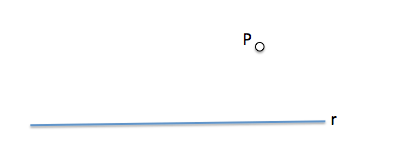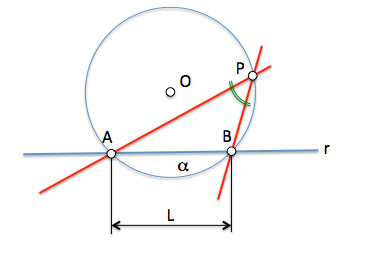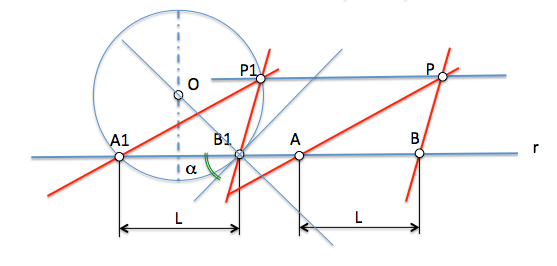# Arco able on a segment : Solución [I]Let the solution to proposed problem Application of arc able, that we proposed with the following statement:

Determine two lines that are based on a point P outside a line r, an angle formed between "alpha" and cut given to the line as a segment of length "L".

The starting geometry proposed for the problem we see in the following image.The point "P" is external to the line "r". The solution should be allowed to find two points, And the B, on the line "r" so to determine a segment with the length "L" and in turn asked each other the angle form given in the statement.In any pair of points (A1-B1) located on the line r and separated a distance L, can determining an arc capable of alpha degrees (given). Any point Pn observe that arc segment A1B1 where the angle alpha. We can determine one (P1) that is at the same distance from r to point P, (to thereby determine a line parallel ar passing through P and intersects the circumference of the arc capable), The lines will be parallel to sought to determine the point P1 and points A1 and B1.We can therefore from any pair of points on r that are separated a distance L to determine the center O of the arc able, which should be in the perpendicular A1-B1 and perpendicular to a straight line passing through one point (eg B1) forms with r the angle alpha. (see arch construction capable)Thus the problem is reduced to obtain a generic solution that will move to the position requested.

Metric geometry